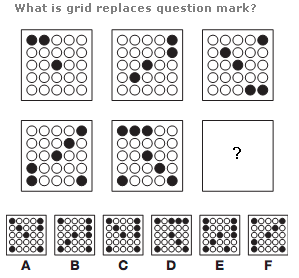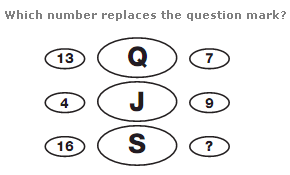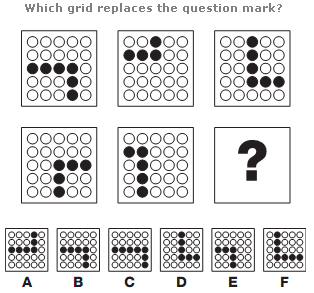# Puzzles - Logical puzzles

Exercise : Logical puzzles
25.:
B Grid
Explanation
:
Working in rows, left to right, top row then bottom, one black circle is added each time, with the positions of the circles moving (1/4) turn clockwise each time.

26.:
6
Explanation
:
In each row of the diagram, the numerical value of the middle letter equals the sum of the left and right hand numbers, subtracting 3.

27.